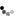# Excel IF function

How to insert the formula for a cell
The values as below
If the cell value is <700. The result is 3600
If the cell value is 700 to 900 the result is 4500
If the cell value is 901 to 2000 the result is 5800

What is the formula if the cell Q5
RESULT CELL IS Q6

You will have to use two if functions in a single formula.

Thank you very much sir

You need to be the querist or approved CAclub expert to take part in this query .

### Similar Resolved Queries